# SEIR修正模型下的武汉地区COVID-19疫情研究与分析Study and Analysis of COVID-19 Pandemic in Wuhan Area Based on SEIR Revision Model

DOI: 10.12677/ORF.2020.103023, PDF, HTML, XML, 下载: 130  浏览: 519  国家自然科学基金支持

Abstract: According to the features of COVID-19, the traditional SEIR infectious disease model was revised and COVID-19 model with the characteristic of transmission was established. Considering the fact that the parameters of the infectious power system are not constant, we divided the disease development of Wuhan area into three stages. Based on the processed data, parameter estimation and model solving were carried out in every step. Combined with the threshold analysis, the changes in model parameters were well explained. With disturbance analysis, the importance of isolation to the scale of this pandemic was confirmed.

1. 绪论

2019年12月底，新型冠状病毒肺炎疫情悄然而至，其波及范围之广，给海内外同胞造成重大影响。在国内，武汉地区首当其冲。自2019年底陆续有病例呈报，其发展态势不断升级。2020年1月22日，湖北省启动突发公共卫生事件II级应急响应。1月23日10时起，武汉市公交，地铁等交通设施暂停运营。1月24日，湖北省启动I级响应，各地医护人员驰援武汉，火神山医院开始投入建设。2月12日起，湖北省确诊标准发生变化，临床诊断被纳入确诊。截止4月7日，武汉市累计报告新冠肺炎确诊病例50,008例，累计出院46,991例，累计病亡2572例。次日4月8日起，离汉离鄂通道管控解除。

2. 数据来源与假设

2.1. 数据来源与说明

2020年1月10日至2020年1月19日武汉地区COVID-19疫情累计确诊人数、死亡人数及治愈人数来源于中华人民共和国国家卫生健康委员会 。此后日期的数据来源于湖北省卫生健康委员会  和中华人民共和国国家卫生健康委员会。通报数据中只包含研究时间阶段内完整的累计确诊人数以及部分死亡人数与治愈人数。

2.2. 基本假设

3. 模型建立

3.1. 三阶段建模

3.2. 建立SEIHCD传染病模型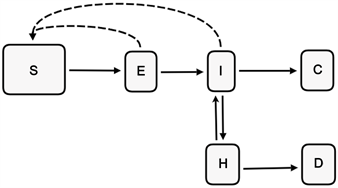Figure 1. Infectious power system of COVID-19

$\frac{\text{d}S\left(t\right)}{\text{d}t}=-{\lambda }_{1}\frac{S\left(t\right)}{N}\beta E\left(t\right)-{\lambda }_{2}\frac{S\left(t\right)}{N}\beta I\left(t\right)$ (1)

$\frac{\text{d}E\left(t\right)}{\text{d}t}={\lambda }_{1}\frac{S\left(t\right)}{N}\beta E\left(t\right)+{\lambda }_{2}\frac{S\left(t\right)}{N}\beta I\left(t\right)-\alpha E\left(t\right)$ (2)

$\frac{\text{d}I\left(t\right)}{\text{d}t}=\alpha E\left(t\right)+\kappa H\left(t\right)-\theta I\left(t\right)-\gamma I\left(t\right)$ (3)

$\frac{\text{d}H\left(t\right)}{\text{d}t}=\theta I\left(t\right)-\kappa H\left(t\right)-\tau H\left(t\right)$ (4)

$\frac{\text{d}C\left(t\right)}{\text{d}t}=\gamma I\left(t\right)$ (5)

3.3. 模型解释

$S\left(t\right),E\left(t\right),I\left(t\right),H\left(t\right),C\left(t\right)$$D\left(t\right)$ 分别表示易感者，潜伏者，普通感染者，重症感染者，治愈者和死亡者数量，由于这六大群体之间没有重复交叉，则在该传染动力系统中，这六个数量之和为武汉总人口数N，即

$S\left(t\right)+E\left(t\right)+I\left(t\right)+H\left(t\right)+C\left(t\right)+D\left(t\right)=N$ (6)

(1)式左边表示易感者人数的变化，右边表示易感者分别受到潜伏者及普通感染者的感染而减少。 ${\lambda }_{1},{\lambda }_{2}$ 分别表示潜伏者和普通感染者的接触率，由于重症感染者集中救治且严格防护，故假设其接触率为0。 $\beta$ 为病毒传染给易感者使其成为潜伏者的概率。

(2)式左边表示潜伏者人数的变化，潜伏者的增加来源于被感染的易感者，潜伏者的减少是由于转为普通感染者。因此右边两个正项代表受潜伏者和普通感染者感染后潜伏者的增加，负项代表潜伏者转为普通感染者。 $\alpha$ 代表潜伏者转化为普通感染者的单位时间概率。

(3)式左边表示普通感染者人数的变化，(3)式右边第一个正项来源于潜伏者，第二个正项来源于重症感染者的好转，另外两个负项表示普通感染者一部分转为重症感染者，另一部分则被治愈。 $\theta$ 表示普通感染者转化为重症感染者的单位时间概率， $\kappa$ 表示重症感染者转化为普通感染者的单位时间概率， $\gamma$ 表示普通感染者转化为治愈者的单位时间概率。

(4)式左边表示重症感染者人数的变化，(4)式右边第一个正项表示部分普通感染者病情加重故重症感染者增加，另外两个负项表示重症感染者的减少，一部分以单位时间概率 $\kappa$ 转为普通感染者，另一部分以单位时间概率 $\tau$ 死亡。

(5)式左边表示治愈者人数的增加，来源于普通感染者。

$\frac{\text{d}D\left(t\right)}{\text{d}t}=\tau H\left(t\right)$ (7)

4. 模型求解

4.1. 数据预处理

4.1.1. 缺失值H补齐Figure 2. Severe proportion time series (including missing data)

4.1.2. 缺失值E补齐

${E}_{n}=\left({I}_{n+5}+{H}_{n+5}+{C}_{n+5}+{D}_{n+5}\right)-\left({I}_{n}+{H}_{n}+{C}_{n}+{D}_{n}\right)$ (8)

${E}_{n},{I}_{n},{H}_{n},{C}_{n},{D}_{n}$ 分别表示时刻n的潜伏者，普通感染者，重症感染者，治愈者和病亡者的实时数量。由此，潜伏者的数量可以全部推算出。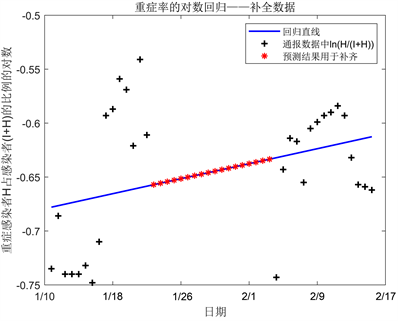Figure 3. Log regression of severe proportion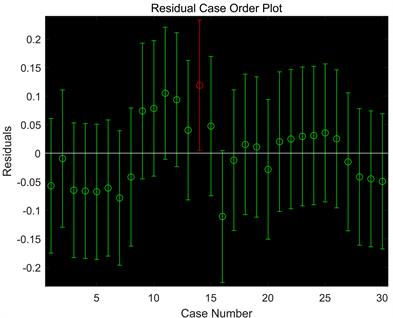Figure 4. Residual time series of log regression based on severe proportion

4.1.3. 确定N的取值

4.1.4. “换帅”骤跳修正

${I}_{n}=A{e}^{Bt}$ (9)

4.2. 参数估计

4.2.1. 估计说明

${\alpha }_{1}=\frac{{\lambda }_{1}}{N},{\alpha }_{2}=\frac{{\lambda }_{2}}{N}$，由公式(1)~(5)得

$\frac{\text{d}S\left(t\right)}{\text{d}t}=-{\alpha }_{1}\beta S\left(t\right)E\left(t\right)-{\alpha }_{2}\beta S\left(t\right)I\left(t\right)$ (10)

$\frac{\text{d}E\left(t\right)}{\text{d}t}={\alpha }_{1}\beta S\left(t\right)E\left(t\right)+{\alpha }_{2}\beta S\left(t\right)I\left(t\right)-\alpha E\left(t\right)$ (11)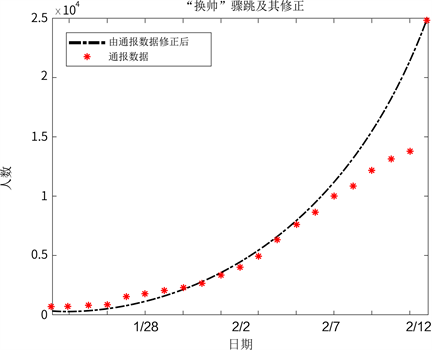Figure 5. Surging data and its correction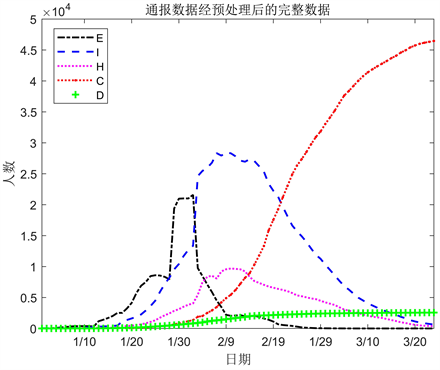Figure 6. Complete data after processing

4.2.2. 经验取值Table 1. Empirical parameter estimate and its basis

4.2.3. 搜索估计

$\mathrm{min}\text{}\underset{t=1}{\overset{s}{\sum }}{\left[I\left(t\right)-{I}^{*}\left(t\right)\right]}^{2}$

4.3. 估计结果

4.3.1. 第一阶段潜伏蔓延期(2020/1/10~2020/1/22)Table 2. Parameter estimation results of the first stage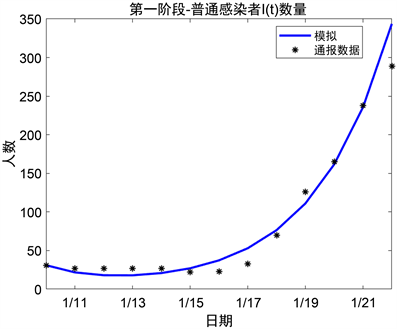Figure 7. Comparison chart between reported data and simulation result of I at the first stage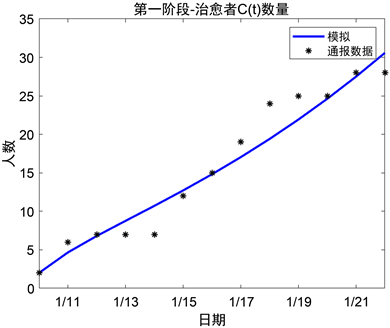Figure 8. Comparison chart between reported data and simulation result of C at the first stage

4.3.2. 第二阶段封城暴发期(2020/1/23~2020/2/12)Table 3. Parameter estimation results of the second stageFigure 9. Comparison chart between reported data and simulation result of I at the second stage

4.3.3. 第三阶段攀升消退期(2020/2/13~2020/4/7)Table 4. Parameter estimation results of the third stage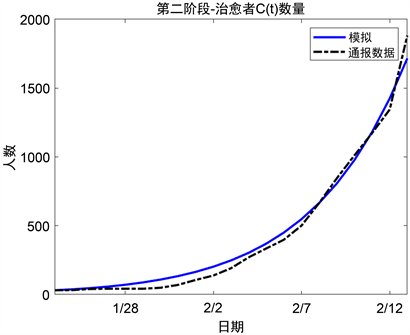Figure 10. Comparison chart between reported data and simulation result of C at the second stage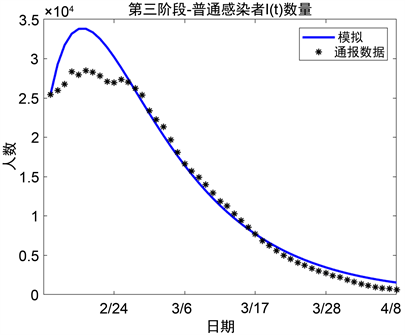Figure 11. Comparison chart between reported data and simulation result of I at the third stage

5. 结果与讨论

5.1. 防控力度对疫情规模的影响Table 5. Comparison of quarantine parameters of the three stages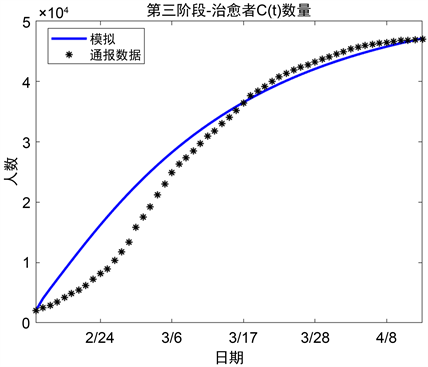Figure 12. Comparison chart between reported data and simulation result of C at the third stage

5.2. 救治水平的分析Table 6. Comparison of curing parameters of the three stages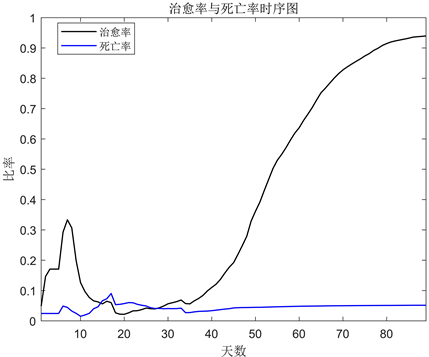Figure 13. Cure rate and death rate time series

5.3. COVID-19疫情阈值分析

5.4. 隔离力度的扰动分析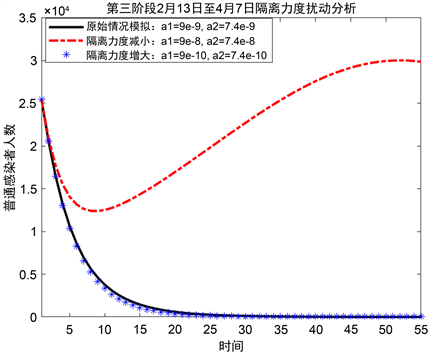Figure 14. Perturbation analysis of quarantine level at the third stage

6. 结语

NOTES

*通讯作者。

  严阅, 陈瑜, 刘可伋, 罗心悦, 许伯熹, 江渝, 程晋. 基于一类时滞动力学系统对新型冠状病毒肺炎疫情的建模和预测[J]. 中国科学: 数学, 2020, 50(3): 385-392.  Wu, J.T., Leung, K. and Leung, G.M. (2020) Nowcasting and Forecasting the Potential Domestic and International Spread of the 2019-nCoV Outbreak Originating in Wuhan, China: A Modelling Study. The Lancet, 395, 689-697. https://doi.org/10.1016/S0140-6736(20)30260-9  姜启源, 谢金星, 叶俊, 数学模型[M]. 北京: 高等教育出版社, 2011.  中华人民共和国国家卫生健康委员会. 新型冠状病毒肺炎疫情通报数据[EB/OL]. http://www.nhc.gov.cn/, 2020-04-07.  湖北省卫生健康委员会. 新型冠状病毒肺炎疫情通报数据[EB/OL]. http://wjw.hubei.gov.cn/fbjd/tzgg/, 2020-04-07.  梁进. 大疫当前, 数学能做什么? [J]. 科学, 2020, 72(2): 57-60.  研究证实新冠病毒引发的COVID-19疫情的平均潜伏期为五天[EB/OL]. https://tech.ifeng.com/c/7uiqBCGw4Uy, 2020-04-18.  腾讯新闻谷雨数据. 武汉封城前后, 人们活跃在哪里?湖北周边均令人揪心[EB/OL]. https://kuaibao.qq.com/s/20200128A0BULY00, 2020-04-18.  曹盛力, 冯沛华, 时朋朋. 修正SEIR传染病动力学模型应用于湖北省2019冠状病毒病(COVID-19)疫情预测和评估[J]. 浙江大学学报(医学版), 2020, 49(2): 178-184.  范如国, 王奕博, 罗明, 张应青, 朱超平. 基于SEIR的新型肺炎传播模型及拐点预测分析[J/OL]. 电子科技大学学报, 2020, 49(3): 369-374. http://www.juestc.uestc.edu.cn/article/doi/10.12178/1001-0548.2020029, 2020-05-11.  Zhao, S., Musa, S.S., Fu, H., et al. (2019) Simple Framework for Real-Time Forecast in a Da-ta-Limited Situation: The Zika Virus (ZIKV) Outbreaks in Brazil from 2015 to 2016 as an Example. Parasites Vectors, 12, Article No. 344. https://doi.org/10.1186/s13071-019-3602-9  尹礼寿. 具有非线性感染力的一类SIR传染病模型的阈值分析[J]. 大学数学, 2016, 32(2): 22-25.  环球网. 钟南山团队曾作出研究: 如管控措施迟5天实施, 疫情规模预估将扩大至3倍[EB/OL]. http://finance.sina.com.cn/china/gncj/2020-03-02/doc-iimxyqvz7253560.shtml, 2020-05-11.